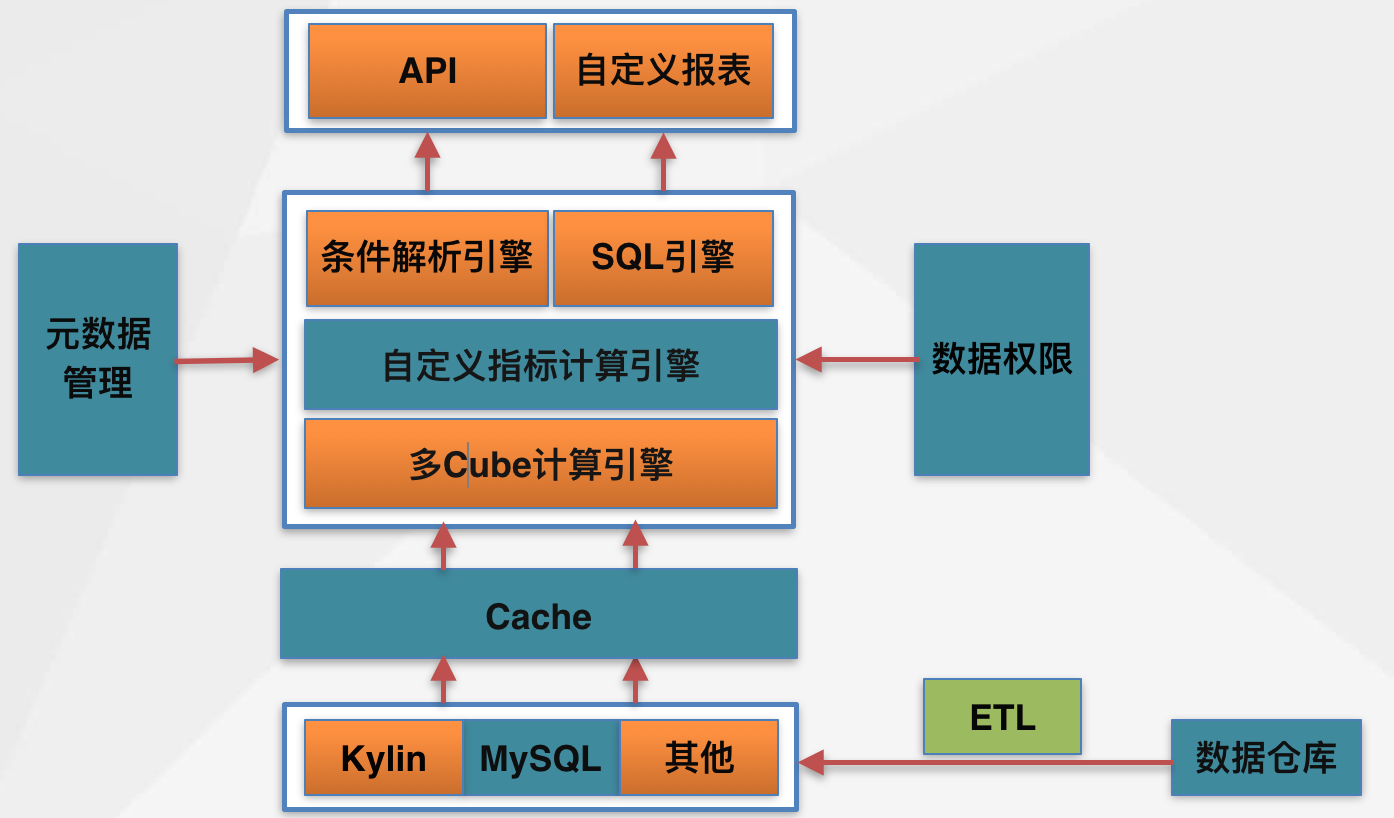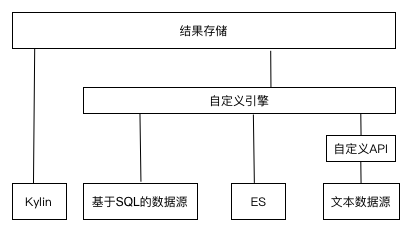• 统一数据源
• SQL生成
• 跨数据源数据聚合
• 自定义计算指标
• 数据权限
• 标准化UI组件，自助生成可视化报表

体系架构具体方案

1. 统一数据源

1. 以什么样的统一方式从数据源获取数据。
2. 不是所有的数据源引擎都能提供OLAP服务和数据聚合的能力，我们需要从上层考虑，去实现数据的聚合、上卷、下钻、切割、自定义计算等功能。2. SQL生成

1. 不是所有的支持SQL的数据源，都支持标准的SQL，同时，支持标准SQL的数据源也会支持带有自身特征的SQL。
2. 根据用户选择的条件、维度和指标，动态生成SQL的核心内容。

1. 这些框架庞大且功能多，适用于我们场景的SQL生成的部分API使用起来过于复杂。
2. 大都是基于标准的ANSI SQL-92，很难个性化地生成我们所需要的SQL。

3. 跨数据源数据聚合

inner join核心代码

private void join(List<Map<String,String>>[] contents,List<Project> sharedList,final int n,int[] rowsStatus,LinkedList<MatchRow> result){
if(this.cubeJoin==1){
throw new java.lang.IllegalArgumentException("left join call leftJoin method,not call join method");
}

if(n<contents.length){
List<Map<String,String>> list = contents[n];
for(int k=0;k<list.size();k++) {
boolean equal = true;
if(n!=0) {
Map<String, String> prev = contents[n - 1].get(rowsStatus[n - 1]);
Map<String, String> cur = list.get(k);

for (Project proj : sharedList) {
String key = proj.fieldName.toUpperCase();
if (key.matches("^\\d+\$") || key.equals("*")) {
key = "_";
}
key = proj.isCompanion() ? key + proj.getFactId() : key;
String prevValue = prev.get(key);
String curValue = cur.get(key);
if (prevValue == curValue) {
continue;
}

if (prevValue == null || curValue == null || !prevValue.equals(curValue)) {
equal = false;
break;
}
}
}
if (equal) {
rowsStatus[n] = k;
if(n==contents.length-1){//last dataset match
MatchRow mr = new MatchRow();

List<MatchRow.DatasetRow> tmp = new ArrayList<>();
for(int i=0;i<rowsStatus.length;i++){
MatchRow.DatasetRow dr = new MatchRow.DatasetRow();
dr.setDatasetIndex(i);
dr.setRowIndex(rowsStatus[i]);
}

}else{
join(contents,sharedList,n+1,rowsStatus,result);
}
}
}
}
}

• contents参数表示每个数据源里面的结果集。
• sharedList表示关联的字段。
• n和rowsStatus是回溯算法记录状态用的。
• result里面包含的是符合join条件的记录。
• MatchRow里面记录的是一个数据源里面的某一行与其余的数据源里面的那一行是相等的，记录的是下标号。

left outer join核心代码

private boolean leftJoin(List<Map<String,String>>[] contents,List<Project> sharedList,final int n,int[] rowsStatus,LinkedList<MatchRow> result){
boolean leftJoinMatch = false;
if(n<contents.length){
List<Map<String,String>> list = contents[n];

for(int k=0;k<list.size();k++) {
boolean equal = true;
if(n!=0) {
//in left join,compare with the first dataset.
Map<String, String> prev = contents.get(rowsStatus);
Map<String, String> cur = list.get(k);

for (Project proj : sharedList) {
String key = proj.fieldName.toUpperCase();
if (key.matches("^\\d+\$") || key.equals("*")) {
key = "_";
}
key = proj.isCompanion() ? key + proj.getFactId() : key;
String prevValue = prev.get(key);
String curValue = cur.get(key);
if (prevValue == curValue) {
continue;
}

if (prevValue == null || curValue == null || !prevValue.equals(curValue)) {
equal = false;
break;
}
}
}
if (equal) {
leftJoinMatch = true;
rowsStatus[n] = k;
if(n==contents.length-1){//last dataset match
MatchRow mr = new MatchRow();

List<MatchRow.DatasetRow> tmp = new ArrayList<>();
for(int i=0;i<rowsStatus.length;i++){
MatchRow.DatasetRow dr = new MatchRow.DatasetRow();
dr.setDatasetIndex(i);
dr.setRowIndex(rowsStatus[i]);
}
}else{
//if next dataset is not match,use the next's next...
for(int loopFlag=n+1;loopFlag<rowsStatus.length;loopFlag++){
boolean match = leftJoin(contents,sharedList,loopFlag,rowsStatus,result);
if(match){
break;
}
rowsStatus[loopFlag]=-1;
if(loopFlag==contents.length-1){
MatchRow mr = new MatchRow();

List<MatchRow.DatasetRow> tmp = new ArrayList<>();
for(int i=0;i<rowsStatus.length;i++){
MatchRow.DatasetRow dr = new MatchRow.DatasetRow();
dr.setDatasetIndex(i);
dr.setRowIndex(rowsStatus[i]);
}
}
}
}
}
}

}
return leftJoinMatch;
}

1. left outer join的数据源匹配逻辑是当第一个数据源与第二个数据源没有匹配的时候，会继续与第三个数据源进行匹配操作，原因是数据源的顺序导致了不匹配，继续往下匹配就可以避免这个问题。
2. 行与行做相等操作的时候，右边没有匹配行的时候，左边的行继续保留，这个是left outer join的逻辑决定的。

4. 自定义计算指标

1. 数据源引擎不支持数据的混合运算或有特殊逻辑的数据处理。
2. 结果数据跨数据源。

1. 通过Java里面的ScriptEngine进行封装来实现数据列的混合运算，不需要自己再去写编译程序解析。对于特殊的数据处理，例如同环比这样的特殊指标，需要单独定义接口，让实现类继承改特定接口，实现类是一个特殊的指标，它需要进行多次数据查询，将最终的结果通过ScriptEngine进行运算。
2. 第二个问题是在上文中“跨数据源数据聚合”的基础上实现的，数据聚合后通过ScriptEngine做最后的处理。

5. 数据权限的问题

1. 使用UPM控制报表的可查看权限。公司推荐使用UPM来控制权限，不过UPM也具有一定的局限性，即只能够判断用户或者组织是否满足某个权限，而不能满足获取部分权限数据的需求，例如某个用户对某个权限只拥有一部分权限，那他就无法提供具体数据。但是UPM可以提供是否的权限，所以报表的可查看权限可以使用UPM来控制，这样可以节约大量的工作。
2. 使用默认任何人都有权限的机制。通过使用默认有权限的这个机制可以大大减少权限数据。需要鉴权的那些维度和指标采用默认无权限的机制，这样两种方案结合，可以最大限度地减少权限数据。
3. 通过走审批流机制自助申请。通过审批流机制可以让用户走自助申请，大大节约权限数据的维护人力成本。